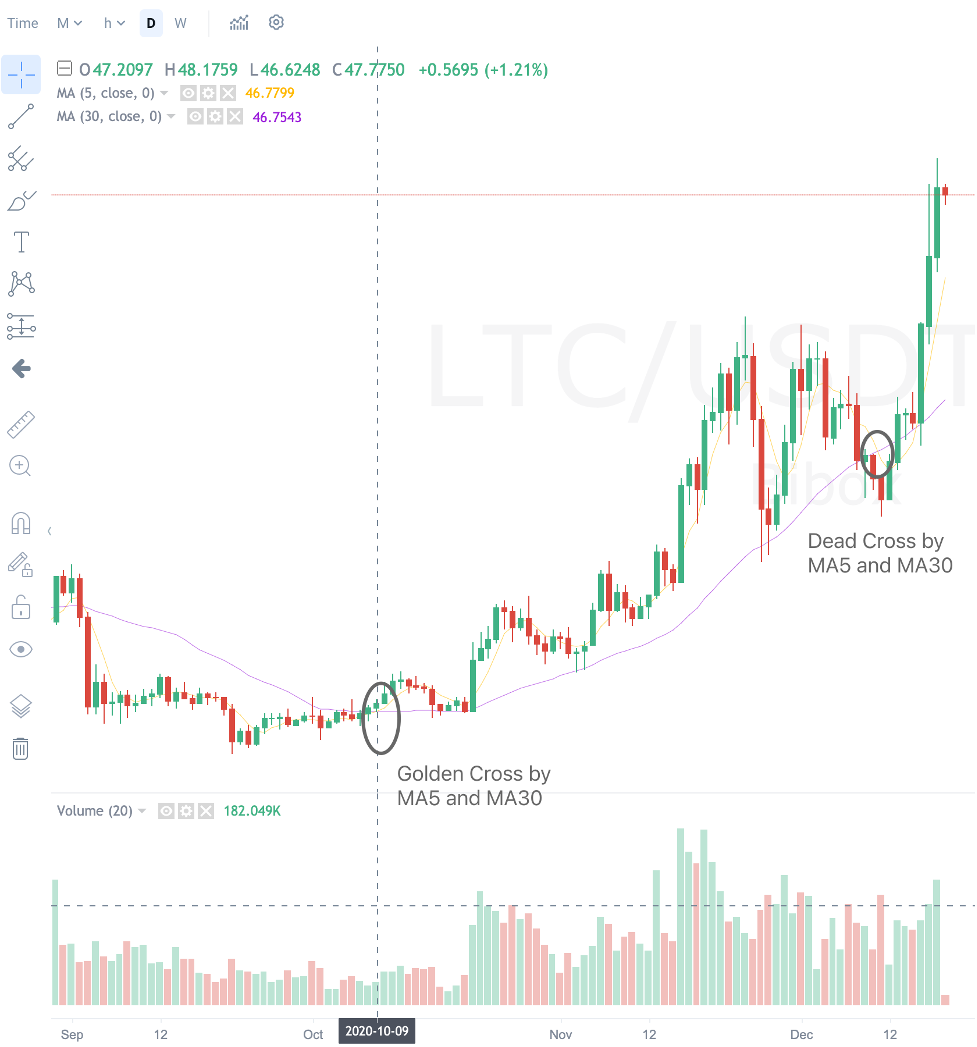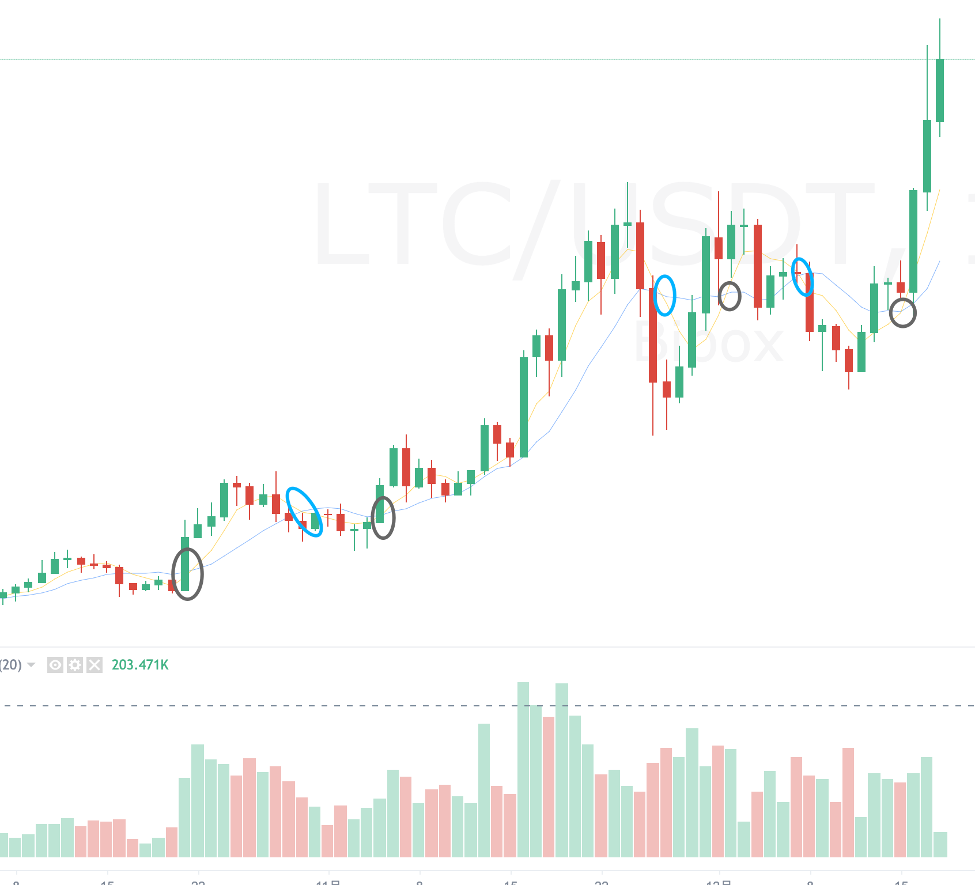# Golden Cross and Dead Cross - A Simple but Profitable Strategy in Cryptocurrency Market

Author: Queent, Created: 2020-12-19 13:56:41, Updated:

Moving average is the average of all the priced points used. For example, the last five closing prices of a token are 32USDT, 34USDT, 35USDT,34USDT,36USDT. The five-day moving average of these closes would be:

（32+34+35+34+36）/5= 34.2 USDT A more general formular is: (P1+P2+P3+P4+P5+…+Pn)/N Where P1 is the most recent price P2 is the second most recent price and so on Pn is the oldest price N is the number of data points.

As the newest data is added to the moving average, the oldest data is dropped. Consequently, the average is always moving as the new data is added. For a five-day moving average, each day’s token price contributes 1/5 to the total moving average and for a nine-day moving average, each day will only be 1/9 of the total moving average. Therefore, the longer the moving average, the less effect an individual price will have on it. In other words, the shorter the term of the moving average, the closer it will ‘hug’ the prices. And it would be more sensitive to the recent price action. The negative aspect of a shorter term is that it has a greater potential for whipsaws. On the other hand, a longer-term moving average provide a greater smoothing effect, but are less responsive to recent prices. Please note there is not only moving average on a daily basis, but also moving average on hour bases. With the same principle applied, we can calculate 5-hour moving average, 5-per 6 hours moving average, which uses the five closing prices of a token in a six-hour trading period.

Please note that most moving average systems use closing prices but highs, lows and so on could also be used while calculating the moving average.

One frequently used trading strategy is the double moving average strategy. We can compare the shorter- and longer- term moving average to each other. If the short-term moving average crosses under the longer-term moving average, it would turn the trend down. And we called this down crossover a ‘dead cross’. It is a signal for sell.

When a shorter-term moving average crosses over a long-term moving average, it would turn the trend up. We call this over crossover a ‘golden cross’. It is a signal for buy.

The following graph shows the candle charts, 5-day moving average and 30-day moving average of LTC/USDT. We can see that on Oct. 9, 2020, there is the golden cross. One can buy LTC at 46.81 USDT. As time goes on, on Dec. 10, 2020, the ‘dead cross ‘shows up. One can sell LTC at 78.9069.The total return within this two month would be：78.9069/46.8166-1=66.76%. This is pretty profitable, especially we consider that no leverage is applied at all.

One can chose different time durations for the strategy. The following graph shows the golden cross(black circle in the graph) and dead cross(blue circle int the grap) of MA5 and MA10 (on a daily basis). We can see that this combination is more sensitive to daily price changes and leads to more frequent buying and selling actions.Investors can back test different tokens’ historical data and summarize whether golden cross and dead cross works for the token you want to buy. Buying selecting reasonable time durations and basis, you may apply this strategy for an aid to make transaction decisions.

Bibix now has double moving average trading bot, which apply this strategy automatically to BTC/USDT, ETH/USDT. More pairs would be supported in the future.

If you are interested in experience this trading bot, you may check it out here https://www.bibox.com/v2/strategy_bot

Of course, you are welcomed to apply this strategy while trading. You may join Bibox trading strategy group here: https://t.me/BiboxExchangeEnglish

More